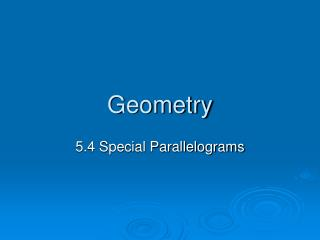DownloadDownload PresentationGeometry

# Geometry

Download Presentation## Geometry

- - - - - - - - - - - - - - - - - - - - - - - - - - - E N D - - - - - - - - - - - - - - - - - - - - - - - - - - -
##### Presentation Transcript

1. Geometry 5.4 Special Parallelograms

2. Set Up a Flow Chart to Fill in as We Go

3. Rectangle • A quadrilateral with four right angles. Why is a rectangle a parallelogram? Both Pairs of Opp. Angles are Congruent

4. Rhombus • A quadrilateral with four congruent sides. Why is a rhombus a parallelogram? Both Pairs of Opp. Sides are Congruent

5. Square (Rhom-tangle Ha! Ha!) • A quadrilateral with four congruent sides and four right angles. Why is a rhombus a parallelogram? Both Pairs of Opp. Sides are Congruent Both Pairs of Opp. Angles are Congruent

6. Review: Rectangles, Rhombuses, and Squares all Share these Properties of a Parallelogram… • Opp. Sides are // • Opp. Angles are congruent • Opp. Sides are congruent • Diagonals Bisect Each Other In addition, rectangles, rhombuses, and squares all have their own special properties. These are the focus of this lesson.

7. Theorem: The diagonals of a Rectangle are Congruent Draw two congruent intersecting lines that bisect each other. Connect the corners. You drew a rectangle.

8. Theorem: The diagonals of a rhombus are perpendicular. Draw two lines that bisect each other & are perpendicular. Connect the corners. You have drawn a rhombus.

9. Theorem: Each diagonal of a rhombus bisects two angles of the rhombus. Draw a rhombus and its diagonals. You bisected all four angles.

10. Theorem: The midpoint of the hypotenuse of a right triangle is equidistant from all three vertices. Draw a right triangle and put a point at the midpoint of the hypotenuse. Draw a line from that point to the vertex of the right angle. All three distances are equal. .

11. Theorem: If an angle of a parallelogram is a right angle, then the parallelogram is a rectangle. Draw one right angle. Draw the two other sides parallel to the opposite side. You have drawn a rectangle. Why is it a rectangle? Opp. Angles of a Parallelogram Are congruent Parallel lines imply SS Int. angles are supplementary.

12. Theorem: If two consecutive sides of a parallelogram are congruent, then the parallelogram is a rhombus. Draw two congruent sides of an angle. Draw the two other sides parallel to the opposite sides. You have drawn a rhombus. Why is it a rhombus? Opp. Sides of a Parallelogram are congruent.

13. Given Quad. WXYZ is a rectangle. Complete the statements with numbers. Make sure your + and – are clear! 3. If TX = 4.5, then WY = _____. 4. If WY = 3a + 16 and ZX = 5a – 18, then a = _____, WY = _____ and ZX = _____. 5. If m<TWZ = 70, then m<TZW = _____ and m<WTZ = _____. W X T Z Y

14. A B 4 2 1 5 3 D C Given Quad. ABCD is a rhombus. Complete the statements with numbers. 7. If m<4 = 25, then m<5 = _____. 8. If m<DAB = 130, then m<ADC = _____. 9. If m<4 = 3x – 2 and m<5 = 2x + 7, then x = ____, m<4 = ____, and m<5 =____. 11. If m<2 = 3y + 9 and m<4 = 2y – 4, then y = _____, m<2 = _____, and m<4 = ____.

15. M L J K Given Quad. JKLM is a square. Complete the statements with numbers. L M x 14. If JL =18, then MK = _____, JX = _____, and XK = _____. 15. m<MJK = _____, m<MXJ = _____ and m<KLJ = _____. X K J

16. HW • P. 186 (1-11) • P. 187 (1-10) (11-27 Odd) If you forget the theorems, it helps to draw a picture…i.e. draw a rhombus and then its diagonals and see if they are congruent or pependicular.

17. A HW Jumpstart P. 187 # 5-8 5) Diags. Bisect each other X X X X 6) Diags. Are conguent X X 7) Diags. Are Perpendicular X X 8) A diagonal Bisects 2 angles X X